# SAS Visual Modeling (A00-274) Certification Exam Sample QuestionsHere are the sample questions which will help you be familiar with SAS Certified Visual Modeling Using SAS Visual Statistics 8.4 (A00-274) exam style and structure. We encourage you to try our Demo SAS Visual Modeling Certification Practice Exam to measure your understanding of exam structure in an environment which simulates the SAS Interactive Model Building and Exploration Using SAS Visual Statistics 8.4 Certification test environment.

To make your preparation more easy for SAS Interactive Model Building and Exploration Using SAS Visual Statistics 8.4 (A00-274) exam, we strongly recommend you to use our Premium SAS Visual Modeling Certification Practice Exam. According to our survey with certified candidates, you can easily score 85% in your actual SAS Certification exam if you can score 100% in our premium SAS Visual Modeling Certification practice exams.

## SAS A00-274 Sample Questions:

01. Which statement is TRUE regarding a generalized additive model (GAM) in SAS Visual Analytics?
a) GAM assumes a strict linear relationship between the predictors and the response function.
b) The roughness penalty controls the balance between goodness of fit and the roughness of the spline curve.
c) Specification of a spline effect is optional.
d) A larger maximum degrees of freedom for the univariate spline term enforces a less complex fit.

02. Which equation does NOT represent a linear model?
Note: bi are parameters and Xi are variables.
a) y = b0 + b1X1 + b2X2
b) y = b0 + b1X1 + b2X2 + b3(X1X2)
c) y = b0 + b1X1 + (b2/b1)X2
d) y = b0 + b1X1 + b2X1 3

03. Refer to the exhibit from a linear regression model in SAS Visual Statistics.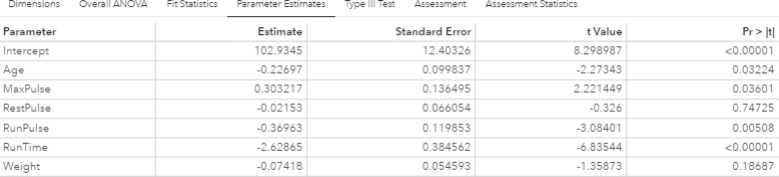Based on the table above and assuming a significance level of 0.05, what can be concluded about the linear regression model?
a) The Intercept is an important predictor of the response.
b) RestPulse is a significant predictor of the response.
c) For one one-unit increase in RunTime, there is an expected increase in the response of 2.6287.
d) For a .03696 unit decrease in RunPulse, there is an expected one-unit increase in the response.

04. Which model does not produce score code?
a) Decision Tree using interactive mode
b) Regression using interaction effects
c) Regression using the group by option
d) Decision Tree using the rapid growth option

05. Refer to the exhibit: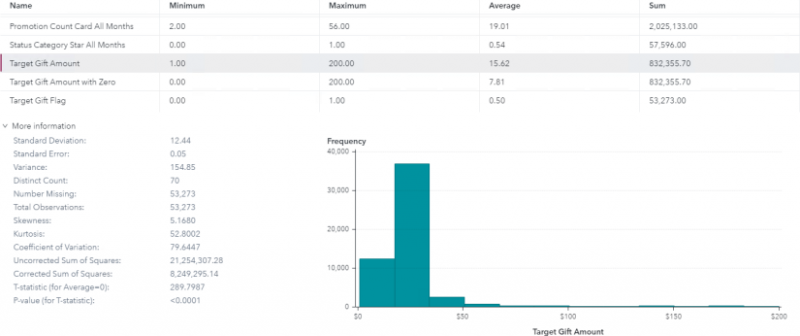Which is the modeling approach that should be used when fitting the Target Gift Amount variable?
a) Linear regression model with Interaction effects.
b) Generalized linear model with a Poisson distribution and Identity link.
c) Generalized linear model with a Normal distribution and Log Link.
d) Logistic regression model.

06. You perform a logistic regression on a multinomial response variable in SAS Visual Statistics that has 3 levels: Small, Medium, Large. "Large" is specified as the event.
Which statement is true?
a) The other levels are grouped into one non-event.
b) An ordinal logistic regression is performed.
c) A multinomial logistic regression is performed.
d) The other levels are offset to account for exposure.

07. Refer to the exhibit: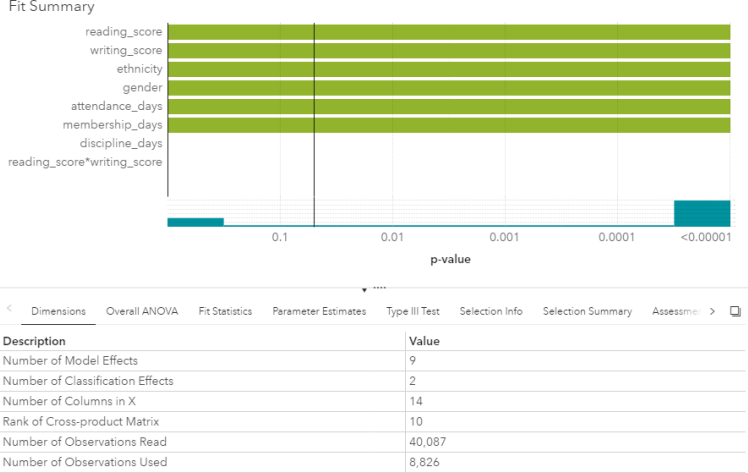Which option was not specified in creating the linear regression model using SAS Visual Statistics?
a) interaction term
b) group-by variable
c) variable selection
d) continuous effects

08. Your company has a dataset that represents global sales. You are a part of a team of analysts that each have responsibility for a certain region of the world. You decide to create a data source filter to suppress every region but yours.
What effect will this have on any new explorations that your teammates create?
a) It will delete all observations that do not match your region.
b) It will have no effect on any observations in the dataset.
c) It will suppress all observations that do not match your region.
d) It will suppress all observations that do not match their corresponding region.

09. In the below nonparametric logistic regression results display, where would you click to get a plot of significant continuous effects?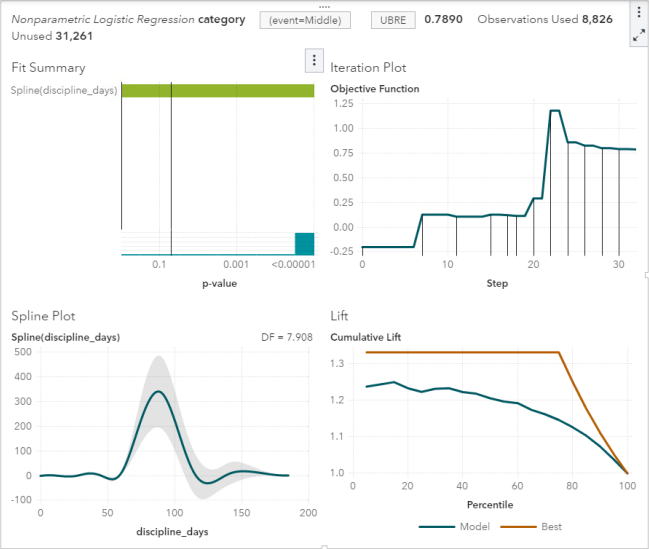Solution: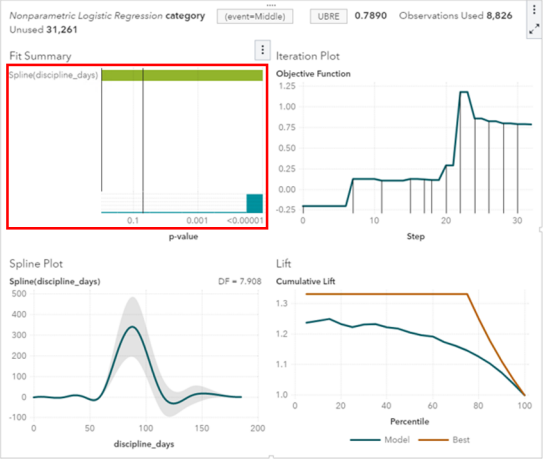Determine whether the given solution is correct?
a) Correct
b) Incorrect

10. You would like to compare multiple models that you've built in SAS Visual Statistics. Which parameters must be the same for all models being compared?
(choose 3)
a) Data Source
b) Assessment Bins
c) Model Type
d) Event Level
e) Response Variable